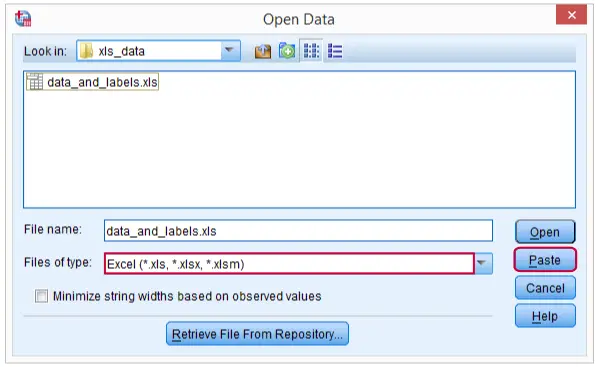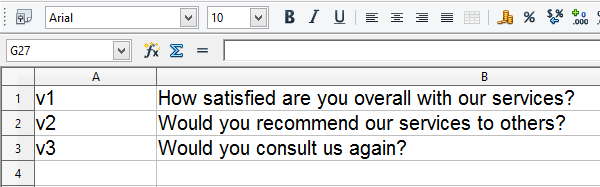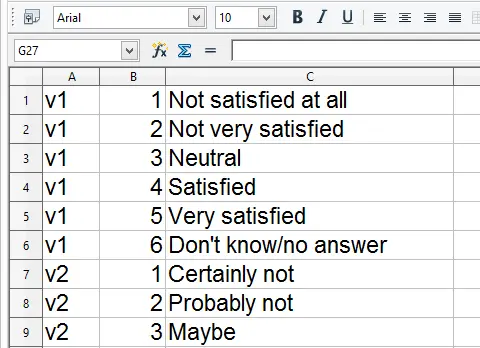SPSS TUTORIALS BASICS ANOVA REGRESSION FACTOR CORRELATION

# Apply Dictionary Information from Excel

## Question

“I have an Excel workbook whose three sheets contain data values, variable labels and value labels. How can I apply the dictionary information from these last two sheets to the SPSS dataset after importing the data values?”

## Option A: Python

A nice and clean option is to have Python read the dictionary information from the Excel sheets. The cell contents can then be inserted into standard `VARIABLE LABELS` and `ADD VALUE LABELS` commands. Running these commands applies the variable labels and value labels to the data values. We'll use data_and_labels.xls for demonstrating this approach.

## 1. Read the Data Values

Reading Excel data values into SPSS is straightforward. We usually paste the required syntax from FileOpenData. The screenshot below shows which options to select.Importing Excel Data into SPSS

## SPSS Syntax for Reading Excel Data

*1. Read data values (pasted syntax from GUI).

GET DATA
/TYPE=XLS
/SHEET=name 'data'
/CELLRANGE=full
/ASSUMEDSTRWIDTH=32767.

## 2. Create Variable Labels Command

Let's first open our workbook and take a look at how the second sheet is structured. As shown in the screenshot below, the first column holds variable names and the second variable labels.SPSS Variable Labels in Excel

Now we'll read this second sheet with Python instead of SPSS. Note that you need to have the SPSS Python Essentials as well as the xlrd module installed first. The syntax below shows how to create the `VARIABLE LABELS` commands as a single (multi line) string. For now we'll just print it for inspection.

## SPSS Python Syntax Example

*2. Create and inspect VARIABLE LABELS commands.

begin program.
import xlrd
varLabCmd = ''
wb = xlrd.open_workbook(xlsPath)
varLabs = wb.sheets()
for rowCnt in range(varLabs.nrows):
rowVals = varLabs.row_values(rowCnt)
varLabCmd += "variable labels %s '%s'.\n"%(rowVals,rowVals.replace("'","''"))
print varLabCmd
end program.

## 3. Create Value Labels CommandSPSS Value Labels in Excel

Remember that Python objects persist over program blocks. We can therefore leave out the first lines of syntax from the previous example. The Excel sheet holding value labels has the same basic structure as the one with variable labels (see screenshot). The main difference is that we'll now insert three pieces of information (variable name, value, value label) into each line. We'll generate our `ADD VALUE LABELS` commands as shown below.

## SPSS Python Syntax Example

*3. Create and inspect ADD VALUE LABELS commands.

begin program.
valLabCmd = ''
valLabs = wb.sheets()
for rowCnt in range(valLabs.nrows):
rowVals = valLabs.row_values(rowCnt)
valLabCmd += "add value labels %s %d '%s'.\n"%(rowVals,rowVals,rowVals.replace("'","''"))
print valLabCmd
end program.

## Running the Python Generated Syntax

If neither of the generated commands require any further tweaking, the only thing left to do is just run them by using `spss.Submit`. The syntax below does so and thus finishes this job.

*4. Run both commands.

begin program.
import spss
spss.Submit(varLabCmd)
spss.Submit(valLabCmd)
end program.

## Option B: Syntax Generating Syntax

Before Python was introduced to SPSS, a different approach was needed for this situation. It comes down to declaring a new (long) string variable and using CONCAT to create lines of syntax as string values. Next, we save the contents of this string variable as a .txt file with an .sps extension and INSERT it.
We don't usually recommend taking this approach but we'll present it anyway for the sake of the demonstration. Some of the commands used by the syntax below are explained in SPSS Datasets Tutorial 1 - Basics and SPSS String Variables Tutorial.

## SPSS Syntax Generating Syntax

*1. Set working directory.

*2. Read data values (pasted syntax from GUI).

GET DATA
/TYPE=XLS
/FILE='data_and_labels.xls'
/SHEET=name 'data'
/CELLRANGE=full
/ASSUMEDSTRWIDTH=32767.

dataset name values.

GET DATA
/TYPE=XLS
/FILE='data_and_labels.xls'
/SHEET=name 'variablelabels'
/CELLRANGE=full
/ASSUMEDSTRWIDTH=32767.

dataset name varlabs.
dataset activate varlabs.

string syntax(a1000).

*4. Create syntax in data window.

compute syntax = concat("variable labels ",rtrim(v1),"'",rtrim(replace(v2,"'","''")),"'.").
exe.

*5. Save variable holding syntax as .sps file.

write outfile 'insert_varlabs.sps'/syntax.
exe.

dataset close varlabs.

*6. Import value labels sheet.

GET DATA
/TYPE=XLS
/FILE='data_and_labels.xls'
/SHEET=name 'valuelabels'
/CELLRANGE=full
/ASSUMEDSTRWIDTH=32767.

dataset name vallabs.
dataset activate vallabs.

string syntax(a1000).

*7. Create syntax in data window.

compute syntax = concat("add value labels ",rtrim(v1)," ",ltrim(str(v2,f3)),"'",rtrim(replace(v3,"'","''")),"'.").
exe.

*8. Save syntax variable as .sps file.

write outfile 'insert_vallabs.sps'/syntax.
exe.

dataset close vallabs.
dataset activate values.

*9. Run both syntax files.

insert file = 'insert_varlabs.sps'.
insert file = 'insert_vallabs.sps'.

*10 Optionally, delete both syntax files.

erase file = 'insert_varlabs.sps'.
erase file = 'insert_vallabs.sps'.

# Tell us what you think!

*Required field. Your comment will show up after approval from a moderator.

# THIS TUTORIAL HAS 9 COMMENTS:

•### By Petri Aaltonen on August 28th, 2016

Dear Ruben

Is there a way to use Python and xlrd (from within SPSS Syntax) to import only specific columns of data from my XLSX data file? I would like to have a row in my XLSX-file with a variable (eg. values 0 or 1) defining whether to import a column to SPSS for further analysis. This is because I have lots of data in the excel sheet that I don't need to analyze in SPSS, and restructuring the excel-file is inconvenient.

•### By Ruben Geert van den Berg on August 29th, 2016

Hi Petri! Sure that's possible. However, I think it'll be much easier to import all data values with the standard pasted SPSS syntax in one go -which doesn't allow excluding variables. Then apply all dictionary information to these data and then just delete whatever you don't need. Or -reversely- delete everything except for what you need with something like

```add files file */keep v4 v12 v35 v51. execute.```

Alternatively, you could have Python read only columns of data values marked with a 1 in some row but then you'll have to somehow pass these values from Python into SPSS -surely possible but harder than the first approach.

•### By Bob Walker on September 20th, 2016

Hi Ruben - I lost your email so am sending this note via your site. I figured it out. On my machine (Windows 10, SPSS V24) I needed the setuptools module. I also had to change this line to read: varLabs = wb.sheets() and not the  index. Works well now!

•### By Ruben Geert van den Berg on September 21st, 2016

Dear Bob, I'm happy to hear things are working now! Generally,  refers to the first sheet,  to the second and so on. With the variable and value labels in sheets 2 and 3, the indices for this example should be  and  unless you removed the first sheet of course.

Best,

Ruben (not "Reuben")!